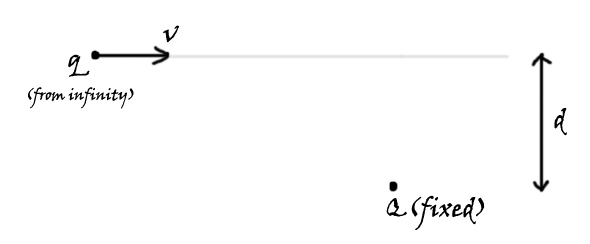# How do they do it?Let a charge $\displaystyle Q$ be held fixed in space. Another charge $\displaystyle q$ of mass $m$ is thrown with a velocity of $\displaystyle v$ from an infinitely far place, as shown in the figure, such that it is at a separation of $\displaystyle d$ initially.

Find the minimum distance (in m) between the two charges.

Details and Assumptions

$\bullet$ $\displaystyle Q = 5\ \mu C$
$\bullet$ $\displaystyle q = 1\ \mu C$
$\bullet$ $\displaystyle d = 3\text{ cm}$
$\bullet$ $\displaystyle v = 2 \text{ m/sec}$
$\bullet$ $\displaystyle m = 1\text{ g}$

×